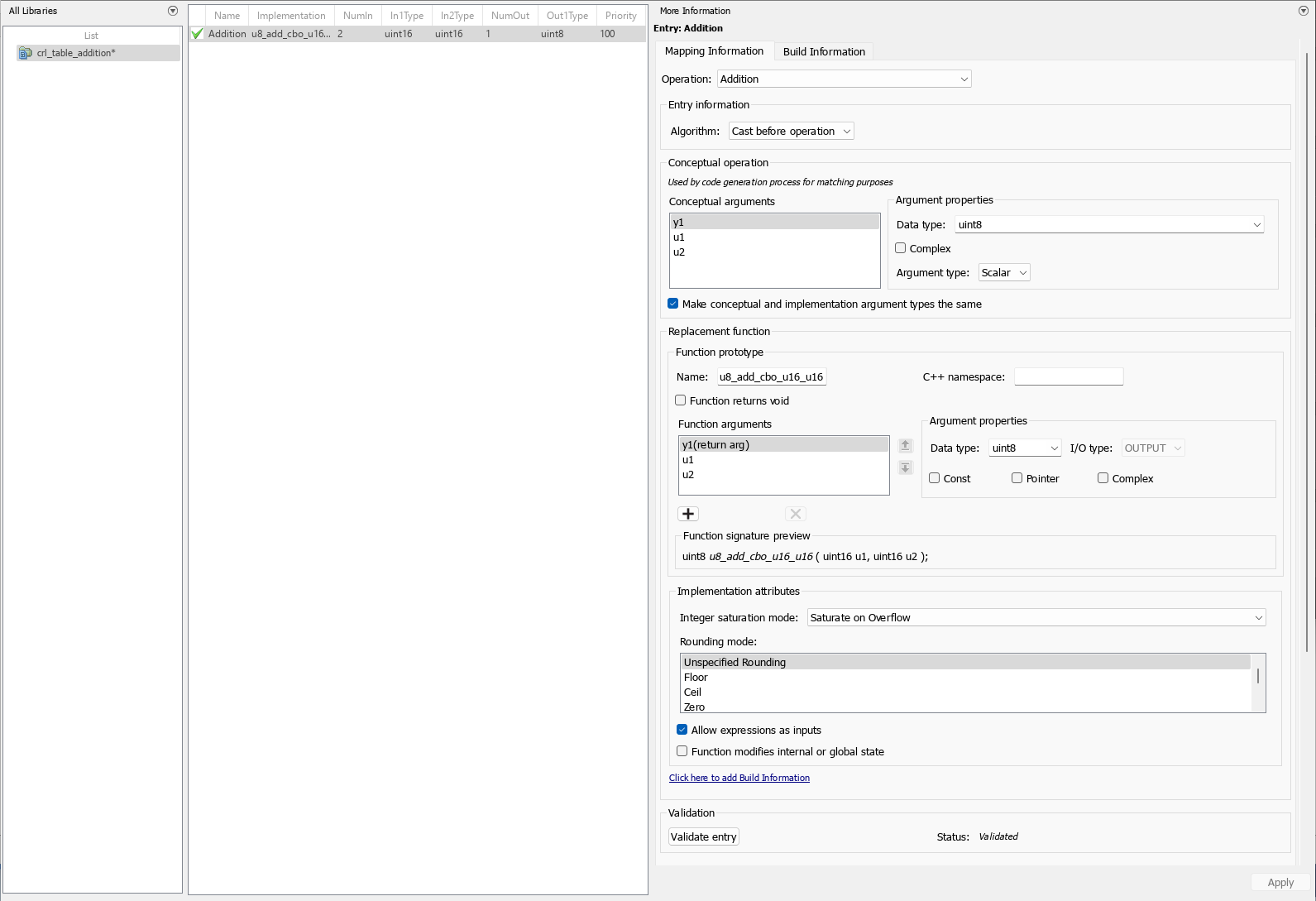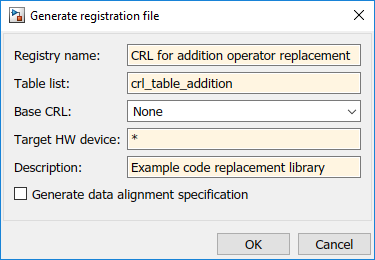## Addition and Subtraction Operator Code Replacement

This example shows how to develop a code replacement library to optimize the performance of addition and subtraction operators by providing information on how to define code replacement for a `addition` operator. To develop a code replacement library use either the interactive or programmatic approach. For more information, see Develop a Code Replacement Library.

### Algorithm Options

When creating a code replacement table entry for an addition or subtraction operator, first determine the type of algorithm that your library function implements.

• Cast-before-operation (CBO), default — Prior to performing the addition or subtraction operation, the algorithm type casts input values to the output type. If the output data type cannot exactly represent the input values, losses can occur as a result of the cast to the output type. Additional loss can occur when the result of the operation is cast to the final output type.• Cast-after-operation (CAO) — The algorithm computes the ideal result of the addition or subtraction operation of the two inputs. The algorithm then type casts the result to the output data type. Loss occurs during the type cast. This algorithm behaves similarly to the C language except when the signedness of the operands does not match. For example, when you add a signed long operand to an unsigned long operand, standard C language rules convert the signed long operand to an unsigned long operand. The result is a value that is not ideal.### Algorithm Classification

During code generation, the code generator examines addition and subtraction operations, including adjacent type cast operations, to determine the type of algorithm to compute the expression result. Based on the data types in the expression and the type of the accumulator (type used to hold the result of the addition or subtraction operation), the code generator uses these rules.

• Floating-point types only

Input 1 Data TypeInput 2 Data TypeAccumulator Data Type Output Data TypeClassification
`double``double``double``double`CBO, CAO
`double``double``double``single`
`double``double``single``double`
`double``double``single``single`CBO
`double``single``double``double`CBO, CAO
`double``single``double``single`
`double``single``single``double`
`double``single``single``single`CBO
`single``single``single``single`CBO, CAO
`single``single``single``double`
`single``single``double``single`
`single``single``double``double`CBO, CAO

• Floating-point and fixed-point types on the immediate addition or subtraction operation

AlgorithmConditions
CBO

One of the following is true:

• Operation type is `double`.

• Operation type is `single` and input types are `single` or fixed-point.

CAOOperation type is a superset of input types—that is, output type can represent values of input types without loss of data.

• Fixed-point types only

AlgorithmConditions
CBO

At least one of the following is true:

• Accumulator type equals output type (```Tacc == Tout```).

• Output type is a superset of input types (`Tacc >= {Tin1, Tin2}`) and accumulator type is a superset of output type (`Tacc >= Tout`).

• Operation does not incur range or precision loss.

CAONet bias is zero and the data types in the expression have equal slope adjustment factors. For more information on net bias, see “Addition” or “Subtraction” in Fixed-Point Operator Code Replacement (for MATLAB® code) or Fixed-Point Operator Code Replacement (for Simulink® models).

In many cases, the numerical result of a CBO operation is equal to that of a CAO operation. For example, if the input and output types are such that the operation produces the ideal result, as in the case of `int8 + int8 —> int16`. To maximize the probability of code replacement occurring in such cases, set the algorithm to cast-after-operation.

### Interactively Develop a Code Replacement Library

1. Open the Code Replacement Tool (crtool), from the MATLAB command line with the following command:

`>>crtool`
2. Create a table.

1. From the crtool context menu, select File > New Table.

2. In the right pane, name the table `crl_table_addition`. Click Apply.

3. Create an entry. From the crtool context menu, select File > New entry > Math Operation.

4. Create entry parameters. In the Function drop-down list, select `Addition`. Algorithm information appears in the crtool. For this example, select the parameter to ```Cast before operation```.

5. Create the conceptual representation. The conceptual representation describes the signature of the function that you want to replace. In the Conceptual function subsection of the crtool, specify the return argument, y1, with the Data Type of `uint8` and the Argument Type of Scalar, the input arguments, u1 and u2 with the Data Type of `uint16` and the Argument Type of Scalar.

6. Create the implementation representation. The implementation representation describes the signature of the optimization function. For this example, to specify that the implementation arguments have the same order and properties as the conceptual arguments, select the Make conceptual and implementation argument types the same check box.

Specify a Name for the replacement function under Function prototype.

7. Specify build information. Click the Build Information tab to open the build requirements pane. Specify the files (source, header, object) that the code generator requires for code replacement. For this example, you do not need to specify build information.

8. Validate and save the table. In the Mapping Information tab, click Validate entry. In the crtool context menu, select File > Save table > Save.9. Register a code replacement library. Registration creates a library composed of the tables that you specify. Select File > Generate registration file. In the Generate registration file dialog box, fill out these fields:To use your code replacement library, refresh your current MATLAB session with the command:

`>>sl_refresh_customizations`

10. Verify the code replacement library. From the MATLAB command line, open the library by using the Code Replacement Viewer and verify that the table and entry are correctly specified. For more information, see Verify Code Replacement Library. Configure your model to use the code replacement library, generate code, and verify that replacement occurs as expected. If unexpected behavior occurs, examine the hit and miss logs to troubleshoot the issues.

### Programmatically Develop a Code Replacement Library

1. Open the programmatic interface from the MATLAB menu by selecting New > Function.

2. Create a table.

1. Create a function with the name of your code replacement library table that does not have arguments and returns a table object. You can use this function to call your code replacement library table.

2. Create a table object by calling `RTW.TflTable`.

```function hTflTable = make_cbo_add_crl_table % Create a function to call the code replacement library table %% Create a table object hTflTable = RTW.TflTable;```
3. Create an entry. Because this example replaces a function, create a code replacement entry in your table by calling the entry function `RTW.TflCOperationEntry`.

```function hTflTable = make_cbo_add_crl_table % Create a function to call the code replacement library table %% Create a table object hTflTable = RTW.TflTable; %% Create an entry hEntry = RTW.TflCOperationEntry; ```
4. Create entry parameters. Because this examples replaces a function, create entry parameters by calling the function `setTflCOperationEntryParameters`.

```function hTflTable = make_cbo_add_crl_table % Create a function to call the code replacement library table %% Create a table object hTflTable = RTW.TflTable; %% Create an entry hEntry = RTW.TflCOperationEntry; %% Create entry parameters hEntry.setTflCOperationEntryParameters(... 'Key', 'RTW_OP_ADD', ... 'EntryInfoAlgorithm', 'RTW_CAST_BEFORE_OP', ... 'ImplementationName', 'u8_add_cao_u16_u16'); ```
5. Create the conceptual representation. The conceptual representation describes the signature of the function that you want to replace. To explicitly specify argument properties, call the function `getTflArgFromString`.

```function hTflTable = make_cbo_add_crl_table % Create a function to call the code replacement library table %% Create a table object hTflTable = RTW.TflTable; %% Create an entry hEntry = RTW.TflCOperationEntry; %% Create entry parameters hEntry.setTflCOperationEntryParameters(... 'Key', 'RTW_OP_ADD', ... 'EntryInfoAlgorithm', 'RTW_CAST_BEFORE_OP', ... 'ImplementationName', 'u8_add_cao_u16_u16'); %% Create the conceptual representation arg = getTflArgFromString(hTflTable, 'y1', 'uint8'); arg.IOType = 'RTW_IO_OUTPUT'; addConceptualArg(hEntry, arg); arg = getTflArgFromString(hTflTable, 'u1', 'uint16'); addConceptualArg(hEntry, arg ); arg = getTflArgFromString(hTflTable, 'u2', 'uint16'); addConceptualArg(hEntry, arg ); ```
6. Create the implementation representation. The implementation representation describes the signature of the optimization function. To specify that the implementation arguments have the same order and properties as the conceptual arguments, call the function `copyConceptualArgsToImplementation`. Add the complete entry to the table by calling the function `addEntry`.

```function hTflTable = make_cbo_add_crl_table() % Create a function to call the code replacement library table %% Create a table object hTflTable = RTW.TflTable; %% Create an entry hEntry = RTW.TflCOperationEntry; %% Create entry parameters hEntry.setTflCOperationEntryParameters(... 'Key', 'RTW_OP_ADD', ... 'EntryInfoAlgorithm', 'RTW_CAST_BEFORE_OP', ... 'ImplementationName', 'u8_add_cao_u16_u16'); %% Create the conceptual representation arg = getTflArgFromString(hTflTable, 'y1', 'uint8'); arg.IOType = 'RTW_IO_OUTPUT'; addConceptualArg(hEntry, arg); arg = getTflArgFromString(hTflTable, 'u1', 'uint16'); addConceptualArg(hEntry, arg ); arg = getTflArgFromString(hTflTable, 'u2', 'uint16'); addConceptualArg(hEntry, arg ); %% Create the Implementation Representation copyConceptualArgsToImplementation(hEntry); %% Add the entry to the table addEntry(hTflTable, hEntry); ```
7. Specify build information. In the entry parameters, specify files (header, source, object) that the code generator needs for code replacement. For this example, build information is not required.

8. Validate and save the customization file. From the MATLAB menu, save this customization file by selecting File > Save. From the command line, validate the code replacement library table by calling it:

`>> hTable = make_cbo_add_crl_table`
9. Register the code replacement library. Registration creates a code replacement library by defining the library name, code replacement tables, and other information. Create a registration file (a new function file) with these specifications:

```function rtwTargetInfo(cm) cm.registerTargetInfo(@loc_register_crl); end function this = loc_register_crl this(1) = RTW.TflRegistry; this(1).Name = 'CRL for addition subtraction operator replacement’; this(1).TableList = {'make_cbo_add_crl_table.m'}; % table created in this example this(1).TargetHWDeviceType = {'*'}; this(1).Description = 'Example code replacement library'; end ```

To use your code replacement library, refresh your current MATLAB session with the command:

`>>sl_refresh_customizations`

10. Verify the code replacement library. From the MATLAB command line, open the library by using the Code Replacement Viewer and verify that the table and entry are correctly specified. For more information, see Verify Code Replacement Library. Configure your model to use the code replacement library, generate code, and verify that replacement occurs as expected. If unexpected behavior occurs, examine the hit and miss logs to troubleshoot the issues.

### Limitations

• When classifying an operation as a CAO operation, the code generator includes the adjacent casts in the expression when the expression involves only fixed-point types. Otherwise, the code generator classifies and replaces only the immediate addition or subtraction operation. Casts that the code generator excludes from the classification appear in the generated code.

• To enable the code generator to include multiple cast operations, which follow an addition or subtraction of fixed-point data, in the classification of an expression, the rounding mode must be `simplest` or `floor`. Consider the expression `y=(cast A)(cast B)(u1+u2)`. If the rounding mode of `(cast A)`, `(cast B)`, and the addition operator (+) are set to `simplest` or `floor`, the code generator takes into account `(cast A)` and ```(cast B)``` when classifying the expression and performing the replacement only.